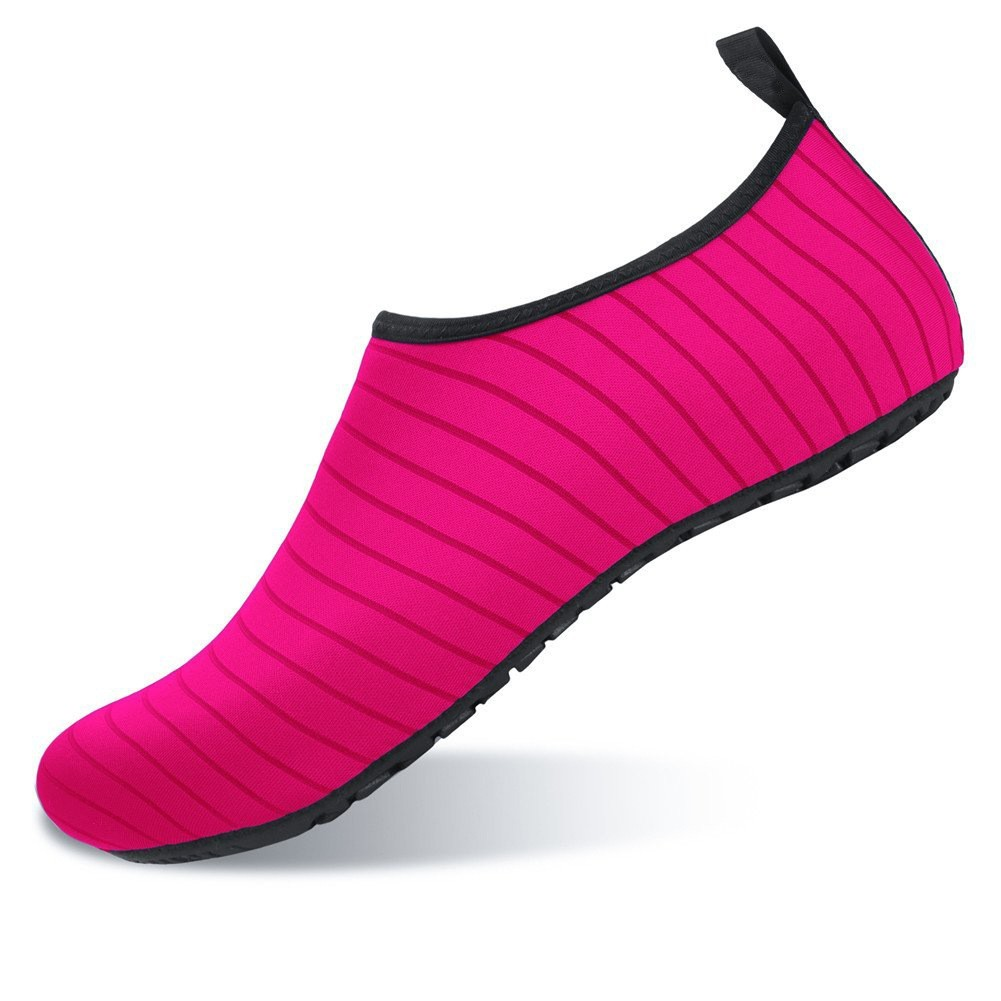# KASUT RENANG WATERSPORT

RM 60.00

Good Quality，I Promise You Will Like！
🌟Ship Time：2-4 days🌟
🌟Ship From : Johor
🌟Size：36-49🌟
🌟Size：EU/UK/CM🌟
🌟Colour : Black
---------------------------------------------------
EU 36 = UK  3  =  23  CM(Foot Length）
EU 37 = UK  4  = 23.5 CM(Foot Length）
EU 38 = UK  5  =  24  CM(Foot Length）
EU 39 = UK  6  = 24.5 CM(Foot Length）
EU 40 = UK  7  =  25  CM(Foot Length）
EU 41 = UK 7.5 = 25.5 CM(Foot Length）
EU 42 = UK  8  =  26  CM(Foot Length）
EU 43 = UK  9  = 26.5 CM(Foot Length）
EU 44 = UK 9.5 =  27  CM(Foot Length）
EU 45 = UK 10  = 27.5 CM(Foot Length）
EU 46 = UK 11  = 28.5 CM(Foot Length）
EU 47 = UK 12  = 28.5 CM(Foot Length）
EU 48 = UK 13  =  29  CM(Foot Length）
EU 49 = UK 14  = 29.5 CM(Foot Length）
---------------------------------------------------
#sandal #shoe #watershoes